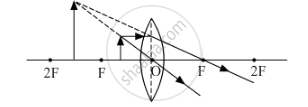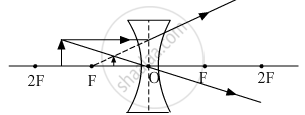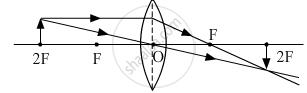# Draw a Ray Diagram in Each of the Following Cases to Show the Formation of Image, When the Object is Placed : (I) Between the Optical Centre and Principal Focus - Science

Diagram

Draw a ray diagram in each of the following cases to show the formation of image, when the object is placed :

(i) between the optical centre and principal focus of a convex lens.
(ii) anywhere in front of a concave lens.
(iii) at 2F of a convex lens.
State the signs and values of magnifications in the above-mentioned cases (i) and (ii).

#### Solution

(i) When an object is placed between the optical center and principal focus of a convex lensSince the image formed is virtual and erect so sign of magnification will be positive. Moreover, the image formed is magnified therefore the absolute value of magnification will be greater than one.

(ii) When an object is placed anywhere in front of a concave lens.Since the image formed is virtual and erect so sign of magnification will be positive. Moreover, the image formed is diminished therefore the absolute value of magnification will be less than one.

(iii) When an object is placed at 2F of a convex lens.Concept: Spherical Mirrors
Is there an error in this question or solution?